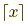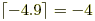index: click on a letter A B C D E F G H I J K L M N O P Q R S T U V W X Y Z A to Z index index: subject areas numbers & symbols sets, logic, proofs geometry algebra trigonometry advanced algebra & pre-calculus calculus advanced topics probability & statistics real world applications multimedia entrieswww.mathwords.com about mathwords website feedback

 Ceiling Function Least Integer Function A step function of x which is the least integer greater than or equal to x. The ceiling function of x is usually written. Sometimes this function is written with reversed floor function brackets, and other times it is written with reversed boldface brackets ]x[ or reversed plain brackets ]x[. Examples:and.See also Floor function (also called greatest integer function)

 this page updated 19-jul-17 Mathwords: Terms and Formulas from Algebra I to Calculus written, illustrated, and webmastered by Bruce Simmons Copyright © 2000 by Bruce Simmons All rights reserved